Open In App

# Binary Decision Diagram

Binary Decision Diagram (BDD) is an effective way to represent the Switching function. It is a Data-Structure used to represent a Boolean Function and can be represented as a compressed form of sets or relations.

### Definition:

A BDD is a set of an Acyclic, Rooted Graph with Directed Edges(Top-to-Bottom), which consist of Decision Node and 2-Terminal Nodes and have a special Node Called ROOT.

A binary decision diagram is a rooted, directed, acyclic graph. Nonterminal nodes in such a graph are called decision nodes; each decision node is labeled by a Boolean variable and has two child nodes, referred to as low child and high child.

BDD is a Shannon cofactor tree:

• f = v fv +v’ fv’  (Shannon expansion)
• Vertices represent decision nodes (i.e. multiplexers) controlled by variables
• leaves are constants “0” and “1”

## Representation of BDD: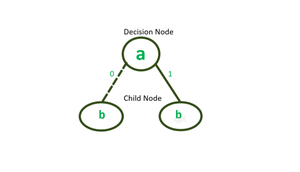Decision and Child Node

• Each Decision Node is labeled by a Boolean variable and has two child nodes called low child and high child.
• The edges from a node to a low child represent, an assignment of variable 0 and for high as 1.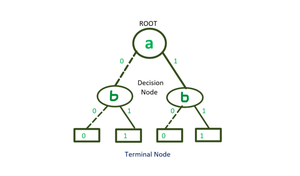Representation of BDD

• Every Decision Node with a variable a and has two child nodes.

A BDD is said to be Ordered if different variables appear in the same order on all paths from the root.

A three-variable function is represented by Truth-table and BDD.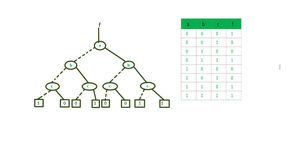BDD of Three-variable function

Variable ordering restriction and reduction rules make (ROBDD) representation canonical

Example:

`f = ac+bc+a'b'c`

Solution-

Step -1: Decomposing the function with respect to variable a

for a’ put a=0 & for a put a=1 in function;

Now, we get-

```f =  a' (b'c'+bc) + a(c+bc)
f =  a'(b'c'+bc) + a(c)```

Step – 2: Decomposing the functions with respect to variable b-

• decompose (b’c’+bc) w.r.to b = b'(c’)+b(c)
• decompose (c) w.r.To b = b'(c)+b(c)

Step – 3: Decomposing the functions with respect to variable c-

• decompose c’ w.r.to c = c'(1)
• decompose c w.r.to c = c(0)
• decompose c’ w.r.to c’ = c'(0)
• decompose c w.r.to c’ = c(1)

We get:

`c'(1)+c(0)      c'(0)+c(1)      c'(0)+c(1)      c'(0)+c(1)`

Note: Here order of variables is a, b, c. But we can take variables in any order.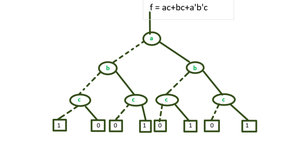BDD of f=ac+bc+a’b’c

### Logical Operation in BDD

Conjunction(∧) – AND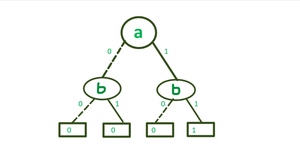BDD of ( a∧ b)

In mathematical notation, we usually write a ∧ b.

In the diagram above for a ∧ b, we indicate the 0 (left) branch with a dashed line and the 1 (right) branch with a solid line. This convention will make it easier to draw diagrams without a lot of intersecting lines.

Disjunction( ∨ ) – OR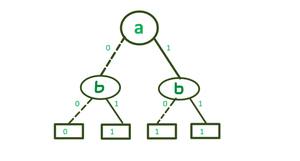BDD of  ( a ∨  b)

In mathematical notation, we usually write a ∨ b.

Negation(⊕ )– NOT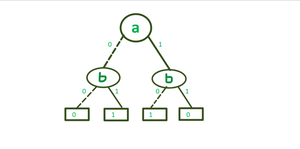BDD of ( a⊕ b )

Exclusive or, which is mathematically often written as a⊕ b.

### Variable Ordering in BDD:

The size of BDD is determined both by the function being represented and the chosen ordering of the variables.

For some functions, the size of a BDD may vary between a linear to an exponential range depending upon the ordering of the variables.

Example:

`f(x1,.......,x2n) = x1x2+ x3x4+.......+x2n-1x2n`

Variable Ordering: x1 < x3 <……<x2n-1< x2 < x4<……< x2n

BDD requires 2n+1 nodes to represent the function.

Variable Ordering: x1<x2<x3<x4<x5<……..<x2n-1<x2n

BDD requires 2n nodes to represent the function.

### Application of BDD:

• BDDs are extensively used in CAD software to synthesize circuits (logic synthesis) and in formal verification.
• In the analysis of Digital Circuit.
• There are several lesser-known applications of BDD, including fault tree analysis, Bayesian reasoning, product configuration, and private information retrieval.